# Civil Engineering - UPSC Civil Service Exam Questions

41.

A propped cantilever is acted upon by a moment M0 at the propped end, what is the prop reaction ?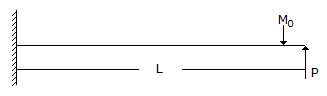A. 0.5 M0 B.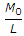C.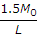D.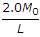Explanation:

No answer description available for this question. Let us discuss.

42.

How are concrete mixers specified ?

 A. By the number of cement bags used in a batch B. By the nominal volume of concrete that can be mixed in a batch C. By the volume of water used D. By the volume of aggregate used

Explanation:

No answer description available for this question. Let us discuss.

43.

In photogrammetric surveying, the image of the top of the hill is 90 mm, from the principal point of the photograph. If the elevation of the top of the hill is 500 m and the flying height is 5000 m above datum, then the relief displacement is

 A. 0.9 mm B. 9 mm C. 90 mm D. 900 mm

Explanation:

No answer description available for this question. Let us discuss.

44.

The phenomenon of decreased resistance of a material due to reversal of stress is called

 A. resilience B. elasticity C. creep D. fatigue

Explanation:

No answer description available for this question. Let us discuss.

45.

A mild steel bar is in three parts, each 20 cm long. The diameters of parts AB, BC and CD are 2 cm, 1 cm and 3 cm respectively.

The bar is subjected to an axial pull of 4t as shown in the given figure. If E = 2 x 106 kg/cm2 and the elongations in the three parts of the bar are Δ1, Δ2 and Δ3 respectively, then the ratio of the greatest to the least of these elongations will be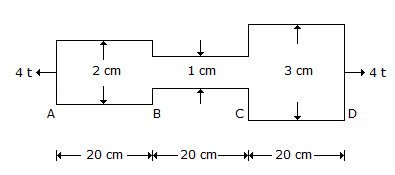A. 9 B. 4 C. 3 D. 2